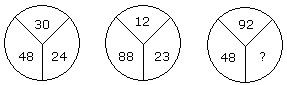# Verbal Reasoning - Character Puzzles - Discussion

### Discussion :: Character Puzzles - Character Puzzles 1 (Q.No.30)

30.

Which one will replace the question mark ?[A]. 60 [B]. 46 [C]. 86 [D]. 75

Explanation:

(30 - 24) x 8 = 48

and (23 - 12) x 8 = 88

Therefore, (92 - 86) x 8 = 48.

 Aasif Javed said: (Dec 2, 2011) In a second why we subtract 23-12 ? There is no similarity to find the value of ? Any one explain please.

 Rahul.N said: (Nov 1, 2014) In the second figure, why do we subtract reverse of the first one. 1. (30-24)8 = 48 but in second one. 2. (23-12)8 = 88. Shouldn't it be (12-23)8 = -88.

 Debasish said: (Jan 18, 2017) If we suppose that the difference part only we can take, in that case "86" can be "98" also, in which the difference if 6 only.

 Sumesh said: (Oct 20, 2019) I can't understand it.